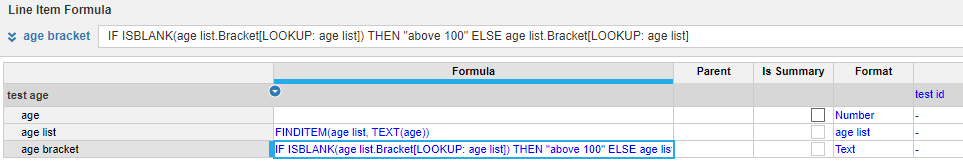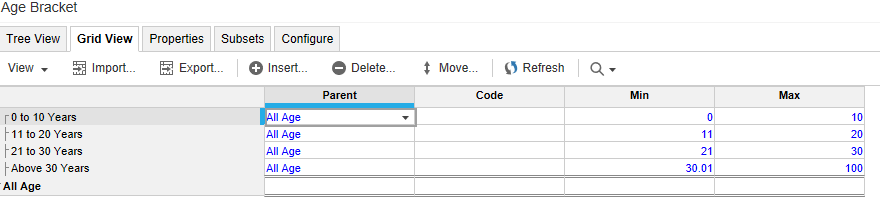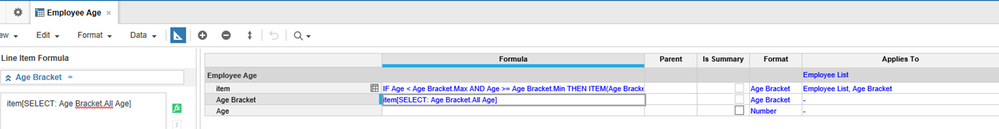# Anaplan function equivalent Excel MATCH functionDear Anaplaners,

What is the Anaplan equivalent function for the "Match" Excel function.

I have a list "Age braket":

0 to 10 years

11 to 20 years

21 to 30 years

Above 30 years

I have 2 line items:

Age : format number

Age range : format: list "Age bracket"

If  "Age" is 25 , I would like to edit a formula in "Age range"  with a result of "21 to 30 years".

I would like to avoid a formula with if "age" < 11 then "0 to 10 years" else....

Emmanuelle

Tagged:

•Hi manuebilly,

I don't like too many nested if statments either.

My preference would be to use mapping instead of formula. I would create a list "age list" of  values from 1 to 100 (or whatever is reasonalble) and then create a property Bracket formated as list Age Bracket. Then mannually map every age value to the Age Bracket list - only takes one time.

In your module you already have 2 line items age (as number) and age bracket (as list)

I would create one more line item:

age list = finditem(age list, text(age))

and then use formula in your age bracket line item:

Age Bracket = age list.Bracket [lookup: age list]

You will have blanks for all values above 100, so I would adjust formula like this:

Age Bracket = if isblank(list.Bracket [lookup: age list]) then "above 100" else list.Bracket [lookup: age list].Hope that helps,

Dmitry

•Hi Manuebilly,

There is also an alternative to generate the item in the "bracket" list. Set minimum and maximum for each item. This will be applicable if the range is more than 100 and may be tedious to maintain mapping of each value to the "bracket" list.In the module, create a line item that would include both the employee list and the "bracket" list. The item summary should be set to "Last Nonblank'Hope this helps,

Jho

•I think you are looking for the FINDITEM function.

Here is one possibility:

Add Code entries to the Age Range list

0 to 10 years - Code = 0

11 to 20 years - Code = 1

21 to 30 years - Code = 2

Above 30 years - Code = 3

Add a Line Item named "Bracket Code" to your module.  Format: Text.  Summary: None

Bracket Code = TEXT(MIN(3, MAX(0, ROUND((Age - 1) / 10, 0, DOWN))))

Age Bracket = FINDITEM(Age Range, Bracket Code)

(This computes the code correctly for 0 to infinity.  You might wish to add an IF condition to return Blank for negative ages).

•Dear all,

thank you very much for your help.

Emmanuelle